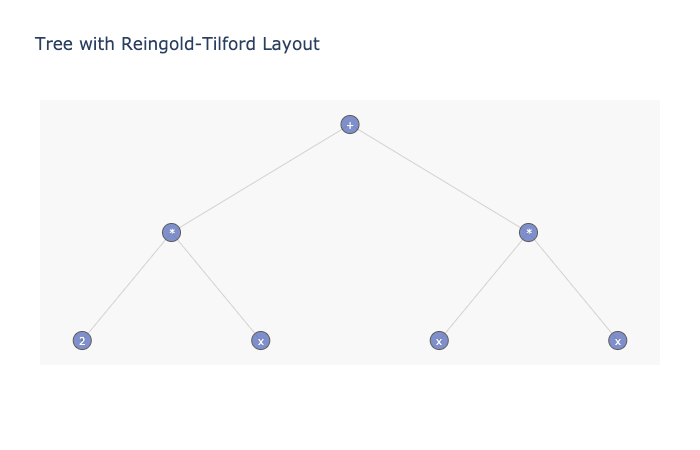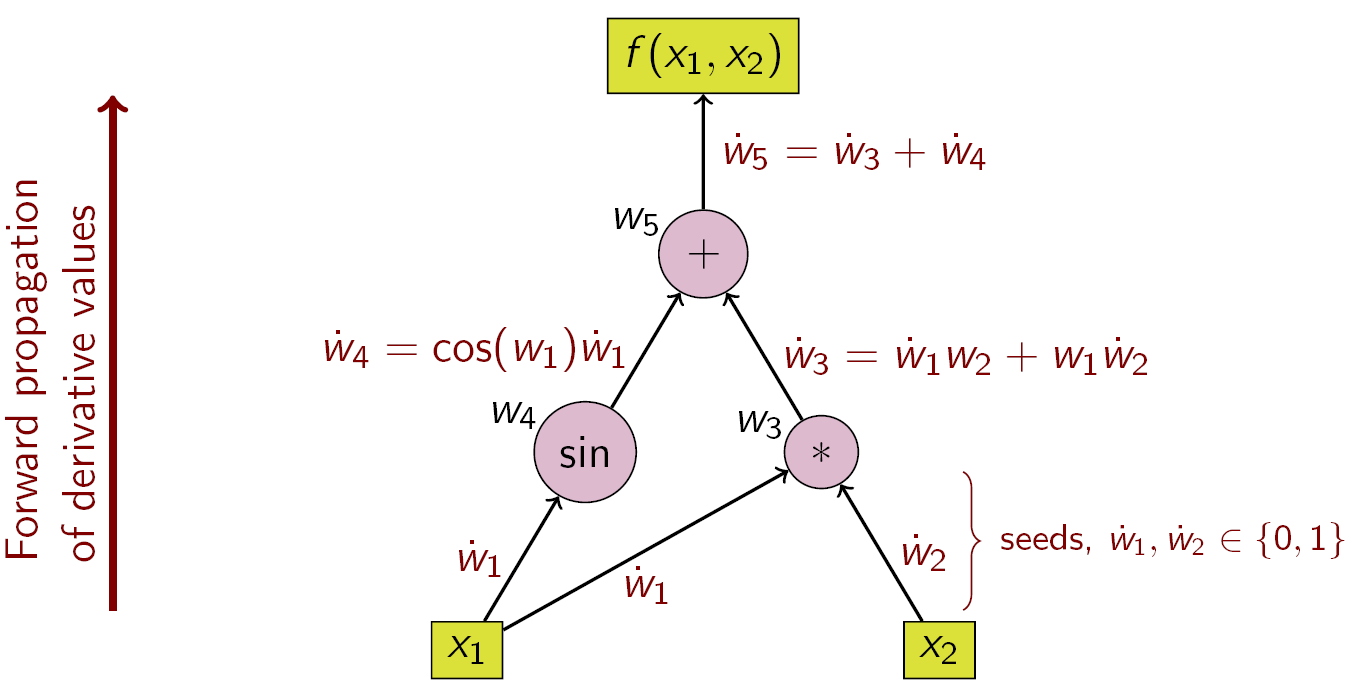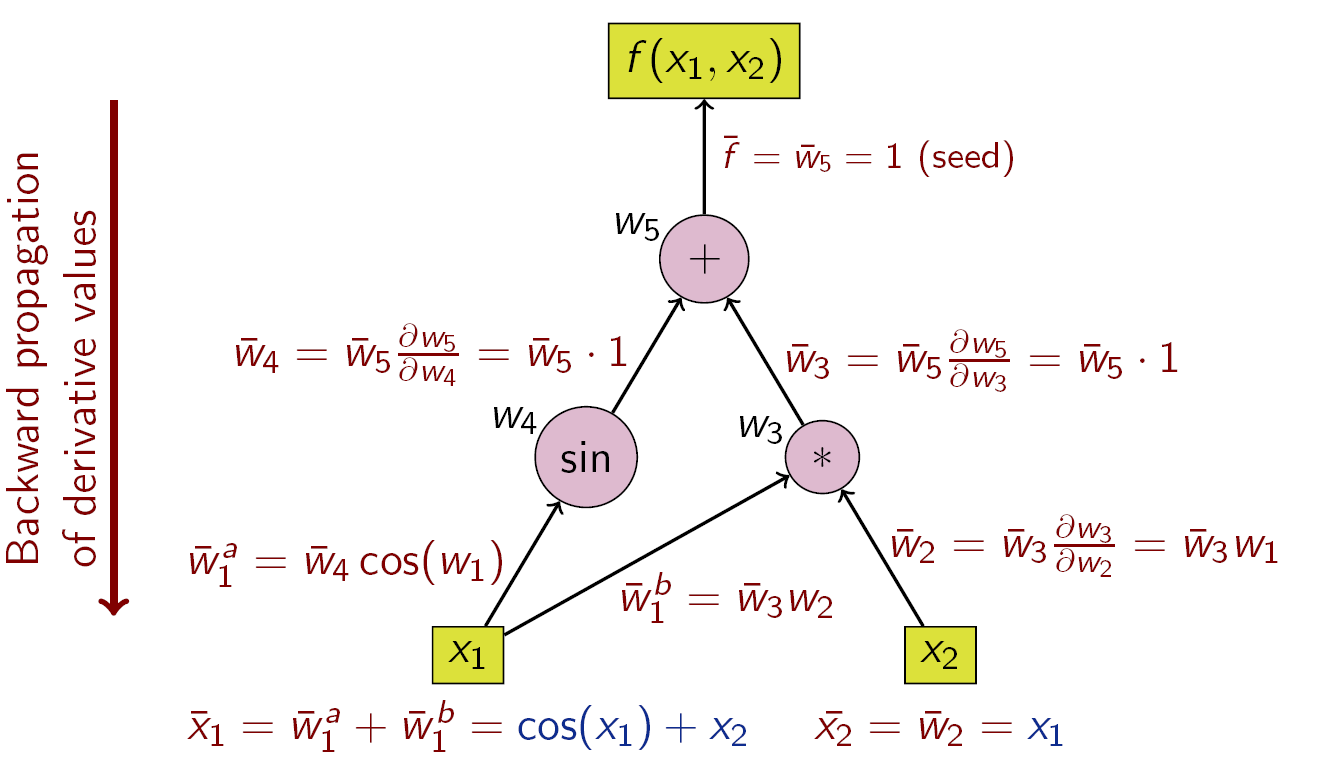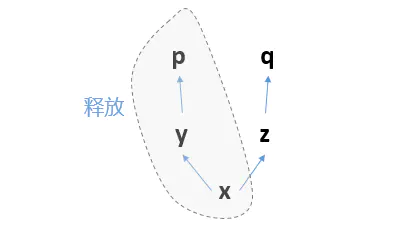# 线性回归

1. 线性回归的基本要素
2. 线性回归模型从零开始的实现
3. 线性回归模型使用pytorch的简洁实现

## 线性回归的基本要素

### 模型

$price=w_{area}*area+w_{age}*age+b$

### 损失函数

$l^{(i)}(w,b)=\frac{1}{2}(\hat y^{(i)}-y^{(i)})^2,\\ L(\mathbf{w},b)=\frac{1}{n}\sum_{i=1}^{n}l^{(i)}(\mathbf{w},b)=\frac{1}{n}\sum_{i=1}^{n}\frac{1}{2}(\mathbf{w}^\top\mathbf{x}^{(i)}-y^{(i)}+b)^2=\frac{1}{2n}\sum_{i=1}^{n}(\mathbf{w}^\top\mathbf{x}^{(i)}-y^{(i)}+b)^2$

### 优化函数 - 随机梯度下降

#### 插曲：什么是梯度下降？

${f_{{x_1}}} = {{\partial y} \over {\partial {x_1}}},{f_{{x_2}}} = {{\partial y} \over {\partial {x_2}}}...{f_{{x_n}}} = {{\partial y} \over {\partial {x_n}}}$

$f(x+\Delta x,y+\Delta y)-f(x,y)=\frac{\part f}{\part x}\Delta x+\frac{\part f}{\part y}\Delta y+o(\rho)$

$\frac{f(x+\Delta x,y+\Delta y)-f(x,y)}{\rho}=\frac{\part f}{\part x}\frac{\Delta x}{\rho}+\frac{\part f}{\part y}\frac{\Delta y}{\rho}+\frac{o(\rho)}{\rho}$

$\lim\limits_{\rho\rightarrow 0}\frac{f(x+\Delta x,y+\Delta y)-f(x,y)}{\rho}=\lim\limits_{\rho\rightarrow 0}(\frac{\part f}{\part x}\frac{\Delta x}{\rho}+\frac{\part f}{\part y}\frac{\Delta y}{\rho}+\frac{o(\rho)}{\rho})\\方向向量：\frac{\part f}{\part l}=\frac{\part f}{\part x}cos\theta+\frac{\part f}{\part y}sin\theta$

• (i)初始化模型参数，一般来说使用随机初始化；
• (ii)我们在数据上迭代多次，通过在负梯度方向移动参数来更新每个参数。

# 1.基本设置

pip3 install xxx -i http://mirrors.aliyun.com/pypi/simple/ --trusted-host mirrors.aliyun.com


import torch
import time

# init variable a, b as 1000 dimension vector
n = 1000
a = torch.ones(n)
b = torch.ones(n)

# define a timer class to record time
class Timer(object):
"""Record multiple running times."""
def __init__(self):
self.times = []
self.start()

def start(self):
# start the timer
self.start_time = time.time()

def stop(self):
# stop the timer and record time into a list
self.times.append(time.time() - self.start_time)
return self.times[-1]

def avg(self):
# calculate the average and return
return sum(self.times)/len(self.times)

def sum(self):
# return the sum of recorded time
return sum(self.times)


timer = Timer()
c = torch.zeros(n)
for i in range(n):
c[i] = a[i] + b[i]
'%.5f sec' % timer.stop()


timer.start()
d = a + b
'%.5f sec' % timer.stop()


# 2.线性回归模型从零开始的实现

# import packages and modules
%matplotlib inline
import torch
from IPython import display
from matplotlib import pyplot as plt
import numpy as np
import random

print(torch.__version__)


## 2.1 生成数据集

$\boldsymbol{y} = \boldsymbol{X}\boldsymbol{w} + b + \epsilon$

# 设置 feature（自变量）的数量
num_inputs = 2
# 设置样本（可探测出的已知的自变量和因变量值）的数量
num_examples = 1000

# 在这里为了生存伪实验数据，先定好参数（weight）的值
true_w = [2, -3.4]
true_b = 4.2

# torch.randn()，正态分布
features = torch.randn(num_examples, num_inputs,
dtype=torch.float32)
labels = true_w * features[:, 0] + true_w * features[:, 1] + true_b
labels += torch.tensor(np.random.normal(0, 0.01, size=labels.size()),
dtype=torch.float32)


randn()的定义如下
def randn(size: _int, generator: Generator, names: Optional[List[Union[str, None]]],
out: Optional[Tensor]=None, dtype: _dtype=None, layout: _layout=strided,
device: Union[_device, str, None]=None, requires_grad:_bool=False) -> Tensor: ...

Returns a tensor filled with random numbers from a normal distribution
with mean 0 and variance 1 (also called the standard normal
distribution).

size是一个可以读入列表的参数，如果输入一些逗号隔开的整数，*号给函数一个讯息，让它把整数整合为一个列表，如果输入两个，第一个就是行数，第二个是列数

lables的初始设置利用了python的广播机制

## 2.2 生成散点图

def use_svg_display():
# 用矢量图显示
display.set_matplotlib_formats('svg')

def set_figsize(figsize=(3.5, 2.5)):
use_svg_display()
# 设置图的尺寸
plt.rcParams['figure.figsize'] = figsize

set_figsize()
plt.scatter(features[:, 1].numpy(), labels.numpy(), 1);  # 加分号只显示图


plt.scatter()必须封入数组，所以只用.numpy()的attribute。将该tensor以NumPy的形式返回ndarray，两者共享相同的底层内存。原tensor改变后会相应的在ndarray有反映，反之也一样。

## 2.3 读取数据

def data_iter(batch_size, features, labels):
num_examples = len(features)
indices = list(range(num_examples))
random.shuffle(indices)  # random read 10 samples
for i in range(0, num_examples, batch_size):
j = torch.LongTensor(indices[i: min(i + batch_size, num_examples)]) # the last time may be not enough for a whole batch
yield  features.index_select(0, j), labels.index_select(0, j)


batch_size = 10

for X, y in data_iter(batch_size, features, labels):
print(X, '\n', y)
break


## 2.4 初始化模型参数

w = torch.tensor(np.random.normal(0, 0.01, (num_inputs, 1)), dtype=torch.float32)
b = torch.zeros(1, dtype=torch.float32)



## 2.5 求导

    Reference: https://www.cnblogs.com/timlong/p/11742535.html
https://www.cnblogs.com/timlong/p/11746848.html
https://en.wikipedia.org/wiki/Automatic_differentiation


### 2.5.2 数值微分法

（其实和下面自动求导的二元数原理没什么差别）

### 2.5.3 符号微分法

$\frac{d}{dx}(f(x)+g(x))=\frac{d}{dx}f(x)+\frac{d}{dx}g(x)$

$\frac{d}{dx}(f(x)*g(x))=g(x)\frac{d}{dx}f(x)+f(x)\frac{d}{dx}g(x)$### 2.5.4 自动求导法

$$a,b\in\mathbb{R}$$,$$\epsilon$$是无穷小量，满足$$\epsilon^2=0$$

$(a+b\epsilon)+(c+d\epsilon)=(a+c)+(b+d)\epsilon$

$(a+b\epsilon)(c+d\epsilon)=ac+(ad+bc)\epsilon$

$f(x+\Delta x)=f(x)+f'(x)\Delta x$

$f(a+b\epsilon)=f(a)+f'(a)b\epsilon\\\Longrightarrow\;f'(a)=\frac{f(a+b\epsilon)-f(a)}{b\epsilon}$

\displaystyle {\begin{aligned}y\;=f(g(h(x)))=f(g(h(w_{0})))=f(g(w_{1}))=f(w_{2})=w_{3}\\w_{0}\;=x\\w_{1}\;=h(w_{0})\\w_{2}\;=g(w_{1})\\w_{3}\;=f(w_{2})=y\end{aligned}}

1. forward accumulation computes the recursive relation $$\displaystyle {\frac {dw_{i}}{dx}}={\frac {dw_{i}}{dw_{i-1}}}{\frac {dw_{i-1}}{dx}}$$ with $$\displaystyle w_{3}=y$$
2. reverse accumulation computes the recursive relation $$\displaystyle {\frac {dy}{dw_{i}}}={\frac {dy}{dw_{i+1}}}{\frac {dw_{i+1}}{dw_{i}}}$$ with $$\displaystyle w_{0}=x$$

#### 2.5.3.1 Forward Mode（前向模式）$\displaystyle {\frac {\partial y}{\partial x}}={\frac {\partial y}{\partial w_{n-1}}}{\frac {\partial w_{n-1}}{\partial x}}={\frac {\partial y}{\partial w_{n-1}}}\left({\frac {\partial w_{n-1}}{\partial w_{n-2}}}{\frac {\partial w_{n-2}}{\partial x}}\right)={\frac {\partial y}{\partial w_{n-1}}}\left({\frac {\partial w_{n-1}}{\partial w_{n-2}}}\left({\frac {\partial w_{n-2}}{\partial w_{n-3}}}{\frac {\partial w_{n-3}}{\partial x}}\right)\right)=\cdots$

$z=f(x_{1},x_{2})\\=x_{1}x_{2}+\sin x_{1}\\=w_{1}w_{2}+\sin w_{1}\\=w_{3}+w_{4}\\=w_{5}$

\displaystyle {\begin{aligned}{\dot {w}}_{1}={\frac {\partial x_{1}}{\partial x_{1}}}=1\\{\dot {w}}_{2}={\frac {\partial x_{2}}{\partial x_{1}}}=0\end{aligned}}“Forward accumulation is more efficient than reverse accumulation for functions f : ℝn → ℝm with m ≫ n as only n sweeps are necessary, compared to m sweeps for reverse accumulation.”

#### 2.5.3.2 Reverse Mode（反馈模式）

$\displaystyle {\frac {\partial y}{\partial x}}={\frac {\partial y}{\partial w_{1}}}{\frac {\partial w_{1}}{\partial x}}=\left({\frac {\partial y}{\partial w_{2}}}{\frac {\partial w_{2}}{\partial w_{1}}}\right){\frac {\partial w_{1}}{\partial x}}=\left(\left({\frac {\partial y}{\partial w_{3}}}{\frac {\partial w_{3}}{\partial w_{2}}}\right){\frac {\partial w_{2}}{\partial w_{1}}}\right){\frac {\partial w_{1}}{\partial x}}=\cdots$## 2.6 定义模型

def linreg(X, w, b):


## 2.7 定义损失函数

$l^{(i)}(w,b)=\frac{1}{2}(\hat y^{(i)}-y^{(i)})^2$

$L_{\mathcal{B}}(w,b)=\frac{1}{|\mathcal{B}|}\sum_{i\in\mathcal{B}}\frac{1}{2}(\hat y^{(i)}-y^{(i)})^2$

def squared_loss(y_hat, y):
return (y_hat - y.view(y_hat.size())) ** 2 / 2


## 2.8 定义优化函数

$(\mathbf{w},b) \leftarrow (\mathbf{w},b) - \frac{\eta}{|\mathcal{B}|} \sum_{i \in \mathcal{B}} \partial_{(\mathbf{w},b)} l^{(i)}(\mathbf{w},b)$

def sgd(params, lr, batch_size):
for param in params:
param.data -= lr * param.grad / batch_size # ues .data to operate param without gradient track


## 2.9 训练

# super parameters init
lr = 0.03
num_epochs = 5

net = linreg
loss = squared_loss

# training
for epoch in range(num_epochs):  # training repeats num_epochs times
# in each epoch, all the samples in dataset will be used once

# X is the feature and y is the label of a batch sample
for X, y in data_iter(batch_size, features, labels):
l = loss(net(X, w, b), y).sum()
# calculate the gradient of batch sample loss
l.backward()
# using small batch random gradient descent to iter model parameters
sgd([w, b], lr, batch_size)
train_l = loss(net(features, w, b), labels)
print('epoch %d, loss %f' % (epoch + 1, train_l.mean().item()))

w, true_w, b, true_b


Reference: https://www.jianshu.com/p/a105858567df

y是一个tensor，那么(y ** 2)/2就是y的对应项相乘再每个项都除以2形成的新tensor，tensor.sum()方法是将tensor的每个项相加，形成一个只有一个元素的tensor，可以看做标量(scalar)RuntimeError: Trying to backward through the graph a second time, but the buffers have already been freed. Specify retain_graph=True when calling backward the first time.

1.更改你的backward函数，添加参数retain_graph=True，重新进行backward，这个时候你的计算图就被保留了，不会报错。

2.你实际根本没必要对一个计算图backward多次，而你不小心多跑了一次backward函数。

# 3. 线性回归用pytorch简洁实现

import torch
from torch import nn
import numpy as np
torch.manual_seed(1)

print(torch.__version__)
torch.set_default_tensor_type('torch.FloatTensor')

1.4.0

# 生成数据集，这里和从零实现的代码是一样的
num_inputs = 2
num_examples = 1000

true_w = [2, -3.4]
true_b = 4.2

features = torch.tensor(np.random.normal(0, 1, (num_examples, num_inputs)), dtype=torch.float)
labels = true_w * features[:, 0] + true_w * features[:, 1] + true_b
labels += torch.tensor(np.random.normal(0, 0.01, size=labels.size()), dtype=torch.float)

# 读取数据集
import torch.utils.data as Data

batch_size = 10

# combine featues and labels of dataset
dataset = Data.TensorDataset(features, labels)

dataset=dataset,            # torch TensorDataset format
batch_size=batch_size,      # mini batch size
shuffle=True,               # whether shuffle the data or not
)

# 定义模型
class LinearNet(nn.Module):
def __init__(self, n_feature):
super(LinearNet, self).__init__()      # call father function to init
self.linear = nn.Linear(n_feature, 1)  # function prototype: torch.nn.Linear(in_features, out_features, bias=True)

def forward(self, x):
y = self.linear(x)
return y

net = LinearNet(num_inputs)
print(net)

LinearNet(
(linear): Linear(in_features=2, out_features=1, bias=True)
)

# 生成多层网络
# ways to init a multilayer network
# method one
net = nn.Sequential(
nn.Linear(num_inputs, 1)
# other layers can be added here
)

# method two
net = nn.Sequential()

# method three
from collections import OrderedDict
net = nn.Sequential(OrderedDict([
('linear', nn.Linear(num_inputs, 1))
# ......
]))

print(net)
print(net)

# 初始化模型参数
from torch.nn import init

init.normal_(net.weight, mean=0.0, std=0.01)
init.constant_(net.bias, val=0.0)  # or you can use net.bias.data.fill_(0) to modify it directly

# 定义损失函数
loss = nn.MSELoss()    # nn built-in squared loss function
# function prototype: torch.nn.MSELoss(size_average=None, reduce=None, reduction='mean')

# 定义优化函数
import torch.optim as optim

optimizer = optim.SGD(net.parameters(), lr=0.03)   # built-in random gradient descent function
print(optimizer)  # function prototype: torch.optim.SGD(params, lr=, momentum=0, dampening=0, weight_decay=0, nesterov=False)

# 训练
num_epochs = 3
for epoch in range(1, num_epochs + 1):
for X, y in data_iter:
output = net(X)
l = loss(output, y.view(-1, 1)) # 讲输出的tensor从左到右，从上到下顺序转化成一个列向量

# result comparision